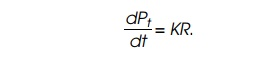Home | | Genetics and Molecular Biology | Proportionality of Ribosome Levels and Growth Rates - Protein Synthesis

# Proportionality of Ribosome Levels and Growth Rates - Protein Synthesis

The dramatic ability of bacteria to grow with a wide variety of rates prompts the question of how they manage to maintain balanced synthe-sis of their macromolecules.

Proportionality of Ribosome Levels and Growth Rates

The dramatic ability of bacteria to grow with a wide variety of rates prompts the question of how they manage to maintain balanced synthe-sis of their macromolecules. In a study of this question, Schaechter, Maaløe, and Kjeldgaard made the discovery that ribosomes are used atconstant efficiency, independent of the cell growth rate. To appreciate their contribution fully, it will be helpful first to examine a related question: what is the average rate of protein synthesis per ribosome? As a first step we will estimate this value using typical cellular parameters, then we will calculate this value more carefully and include the effects of increase in the number of ribosomes during a cell doubling time.

An average bacterial cell with a doubling time of 50 minutes contains about 1 × 10-13 g protein and about 10,000 ribosomes. Approximating the molecular weight of amino acids to be 100,

1 × 10-13 g protein is 1 × 10-13/102 = 10-15 moles amino acid;

10-15 moles amino acid is 6 × 1023× 10-15 = 6 × 108 molecules;

104 ribosomes polymerize these 6 × 108 amino acids in 50 min or 3 ×103sec.

Thus the average rate of protein synthesis per ribosome is 6 × 108/3 × 103= 20 amino acids per second per ribosome. Compared to the typicalturnover number of enzymes, greater than 1,000 per second, this is a low number. We have seen already, however, the process of addition of a single amino acid to the growing polypeptide chain is complex and involves many steps.

To calculate accurately the rate of protein synthesis per ribosome during steady-state growth, we must include the growth of the cells in the calculation. This can be done in the following way. Define αr as the relative rate of synthesis of ribosomal protein, that is,where Pr, is ribosomal protein and Pt is total protein. Let R(t) be the number of ribosomes in a culture at time t. Since ribosome number willthe rate of ribosome synthesis, dR/dt, equals the rate of ribosomal protein synthesis in amino acids per unit time divided by the number of amino acids in the protein of one ribosome, C:Then dPt/dt equals the number of ribosomes times the average elonga-tion rate per ribosome, K. That is,We have the following two expressions for dR/dtwhich yields K =µC/αr, our desired relation. Note that if αr is roughly proportional to the growth rate, as has been found for bacteria except at the slowest growth rates (Fig. 7.24), then the term µ/αr is a constant and hence K, average activity of a ribosome, is independent of the growth rate.

For E. coli B/r growing at 37°, with a doubling time of 48 minutes,= 17 aminoacidsper ribosomepersecond.

This value is close to the elongation rate of polypeptides, showing that most ribosomes in the bacterial cell are engaged in protein synthesis and are not sitting idle.

A measurement of αr at any time during cell growth can be accom-plished by adding a radioactive amino acid to growing cells for a short interval (Fig. 7.25). Then an excess of the nonradioactive form of the amino acid is added and cells are allowed to grow until all the radioac-tive ribosomal proteins have been incorporated into mature ribosomes. The value of αr, is the fraction of radioactivity in ribosomal protein compared to total radioactivity in all the cellular protein. This fraction can be determined by separating ribosomal protein from all other cellular protein by electrophoresis or sedimentation and measuring the radioactivity in each sample.

Study Material, Lecturing Notes, Assignment, Reference, Wiki description explanation, brief detail
Genetics and Molecular Biology: Protein Synthesis : Proportionality of Ribosome Levels and Growth Rates - Protein Synthesis |